Try out our new practice tests completely free!# Quiz 13: Basic Op-Amp Circuits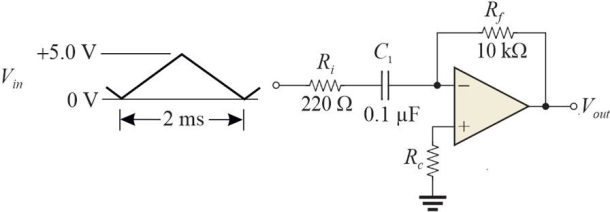Figure 6 -Refer to Figure 6. You observe large decaying oscillations on the output. A likely cause is(Multiple Choice)

C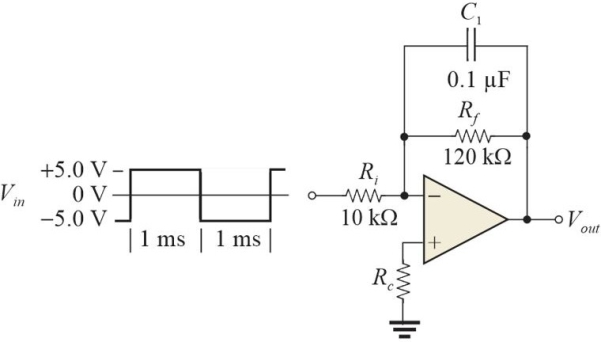Figure 5 The input is a 500 Hz, 10 Vpp square wave centered about 0 V. -Refer to Figure 5. After steady- state conditions are reached, the output will be(Multiple Choice)

D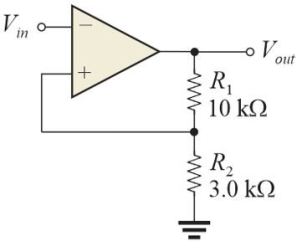Figure 1 Assume that +Vout(max)= +13 V and -Vout(max)= -13 V -Refer to Figure 1. If the input is a 2 Vpp sine wave, the output will be(Multiple Choice)

B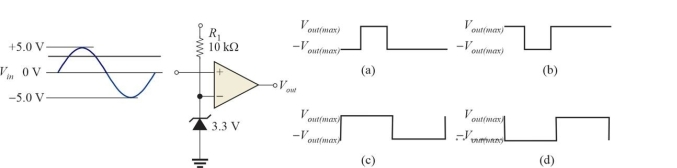Figure 4 The sine wave shown is the input signal. -Refer to Figure 4. The correct output waveform is represented by
(Multiple Choice)Figure 6 -Refer to Figure 6. The output waveform is a
(Multiple Choice)The process of limiting the output of a comparator is called
(Multiple Choice)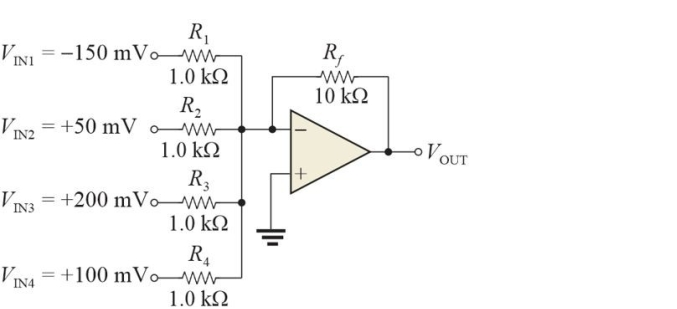Figure 2 -Refer to Figure 2. Assume there is a fault and the output = -14 Vdc. A possible cause is
(Multiple Choice)Figure 1 Assume that +Vout(max)= +13 V and -Vout(max)= -13 V -Refer to Figure 1. If the input voltage is +11 V, the output will be
(Multiple Choice)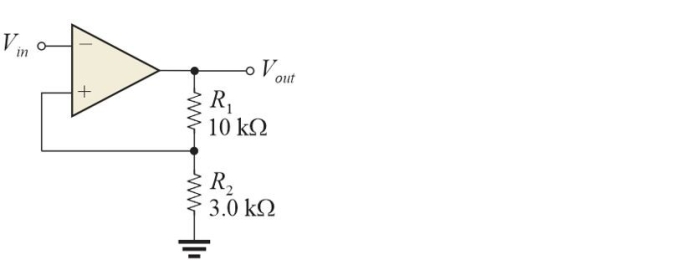Figure 1 Assume that +Vout(max)= +13 V and -Vout(max)= -13 V -Refer to Figure 1. The LTP is at
(Multiple Choice)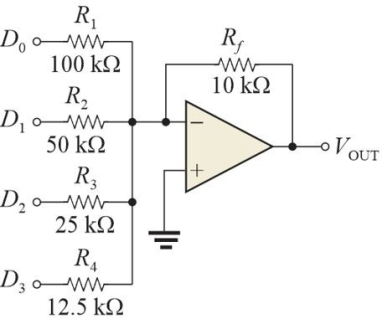Figure 3 -Refer to Figure 3. Assume all inputs are +3.0 V. The output will be
(Multiple Choice)Hysteresis avoids noise by using
(Multiple Choice)A simultaneous (flash)ADC is particularly useful when
(Multiple Choice)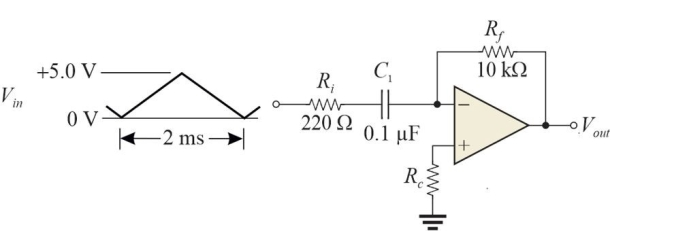Figure 6 -Refer to Figure 6. The primary purpose of Ri is to
(Multiple Choice)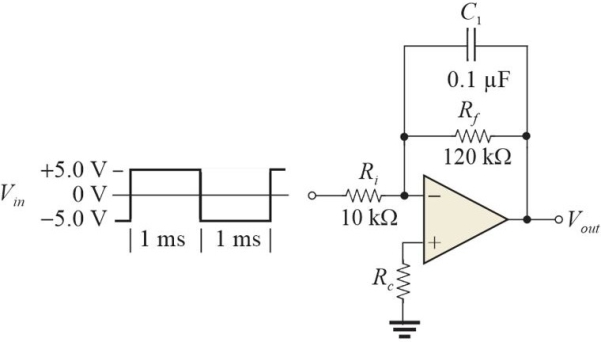Figure 5 The input is a 500 Hz, 10 Vpp square wave centered about 0 V. -Refer to Figure 5. The primary purpose of Rf is to
(Multiple Choice)Figure 5 The input is a 500 Hz, 10 Vpp square wave centered about 0 V. -Refer to Figure 5. Rc is in the circuit to balance the bias currents. A good value for Rc is
(Multiple Choice)If a capacitor is charged with a constant current, the voltage across the capacitor is
(Multiple Choice)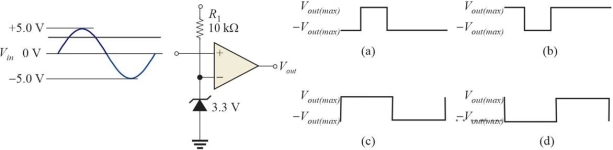Figure 4 The sine wave shown is the input signal. -Refer to Figure 4. If the zener were reversed, the output would
(Multiple Choice)Figure 1 Assume that +Vout(max)= +13 V and -Vout(max)= -13 V -Refer to Figure 1. The UTP is at
(Multiple Choice)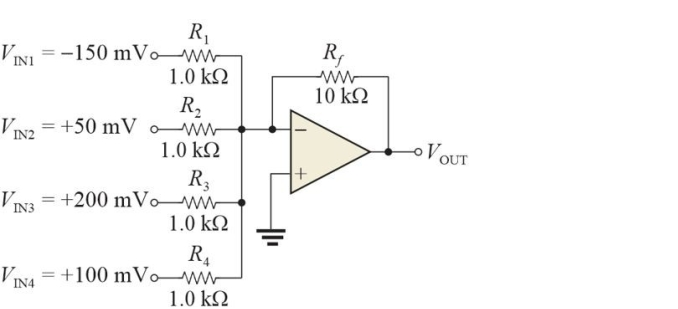Figure 2 -Refer to Figure 2. The current in Rf is
(Multiple Choice)The purpose of a zener diode between the output and inverting input of a comparator is to
(Multiple Choice)## Filters

• Essay(0)
• Multiple Choice(0)